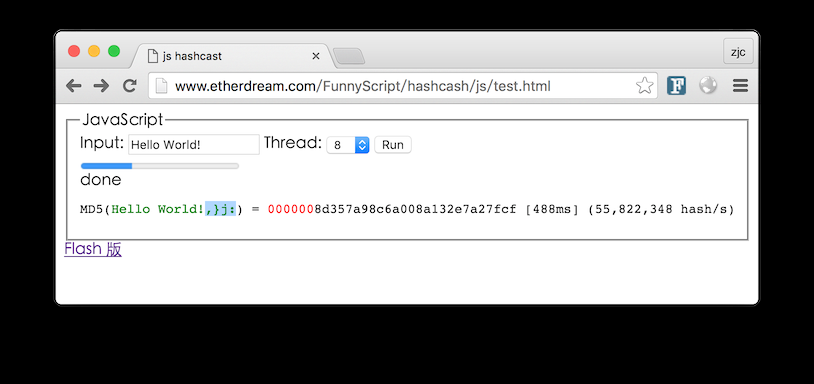# 0x01 如何消耗时间

``````#!cpp
Sleep(1000)
``````

``````#!cpp
for i = 0 to 1000000000
end
``````

``````#!cpp
result = 0
for i = 0 to 1000000000
result = result + i
end
return result
``````

``````#!cpp
MD5(X) == X
``````

``````#!cpp
MD5("问题" + X) == "0000......"
``````

# 0x02 传统应用

Hashcash 早不是新鲜事，曾经在反垃圾邮件中就已使用。

``````#!cpp
Hash(X, Salt) == "000000..."
``````

# 0x03 Web 应用

``````#!cpp
Hash(X, 帖子内容) == "000000..."
``````

``````#!cpp
# 后端 - 分配
session["pow_code"] = rand()

# 前端 - 挖矿
while Hash(X, pow_code) == "000000..."
X = X + 1
end
``````

``````#!cpp
# 前端 - 提交
wait X
submit(..., X)

# 后端 - 校验
if Hash(X, session["pow_code"]) == "000000..."
ok
else
fail
end
``````

# 0x05 致命缺陷

Hashcash 的本质就是跑 hash，这是 GPU 最擅长的。例如著名的 oclHashcat，和 CPU 完全不在一个数量级。

• 硬件瓶颈
• 移植难度
• CPU 算法
• 以暴制暴
• 代码混淆
• 串行模式

# 0x08 串行模式

Hashcash 的原理，决定了它是可以并行计算的。有什么样的算法，是无法并行计算的？

``````#!cpp
function slowhash(x)
for i = 0 to 1000000000
x = hash(x)
end
return x
end
``````

``````#!cpp
# 隐蔽的脚本
Q = rand()
A = slowhash(Q)

submit(Q, A)
``````

``````#!cpp
# 后端 - 分配问题
Q = select_key_from_db()
session["pow_ques"] = Q

# 前端 - 计算问题
A = slowhash(Q)
``````

``````#!cpp
# 前端 - 提交
submit(..., A)

# 后端 - 鉴定
Q = session["pow_ques"]
if A == db[Q]
ok
else
fail
end
``````

# 0x09 演示

https://github.com/EtherDream/proof-of-work-hashcash

http://www.etherdream.com/FunnyScript/hashcash/js/test.html